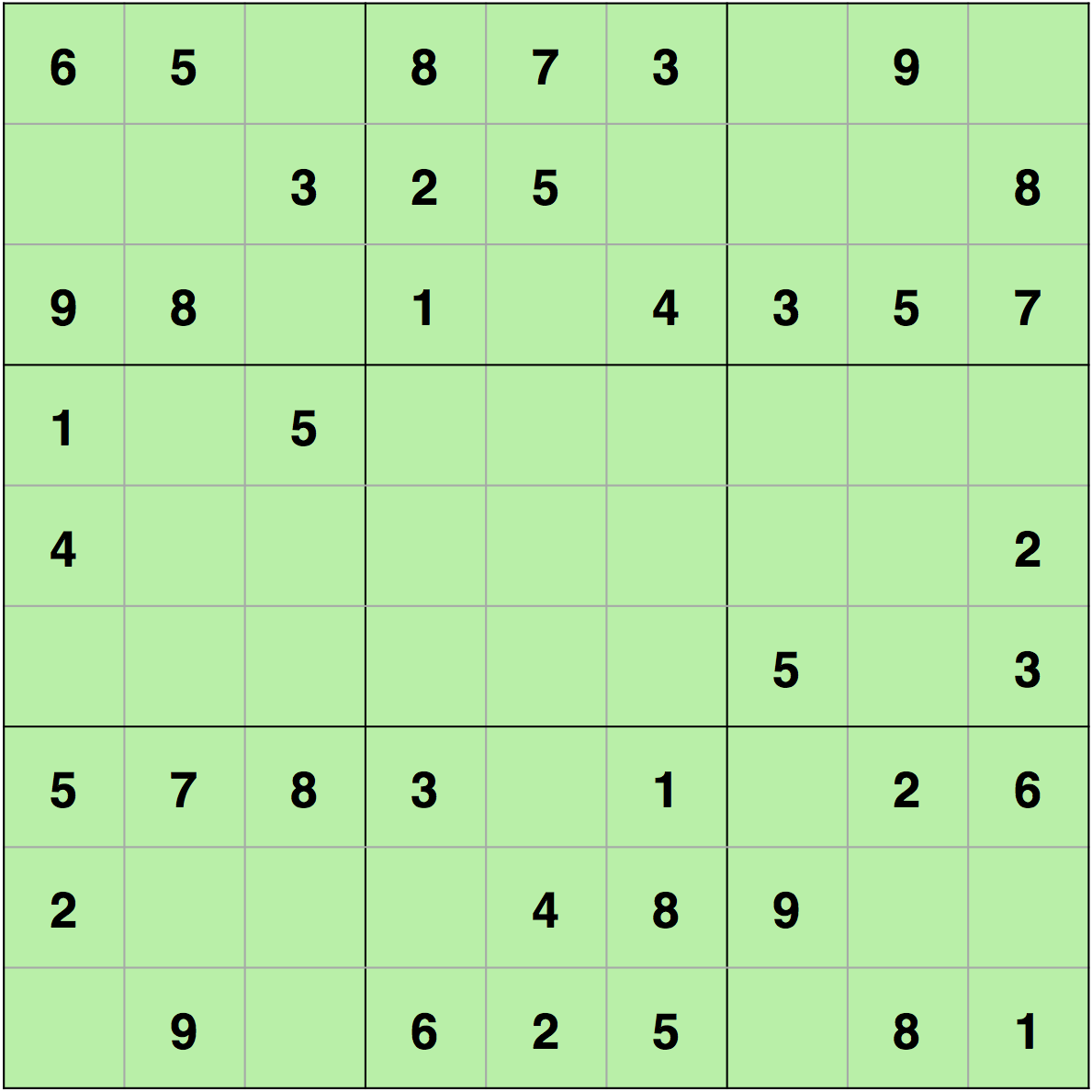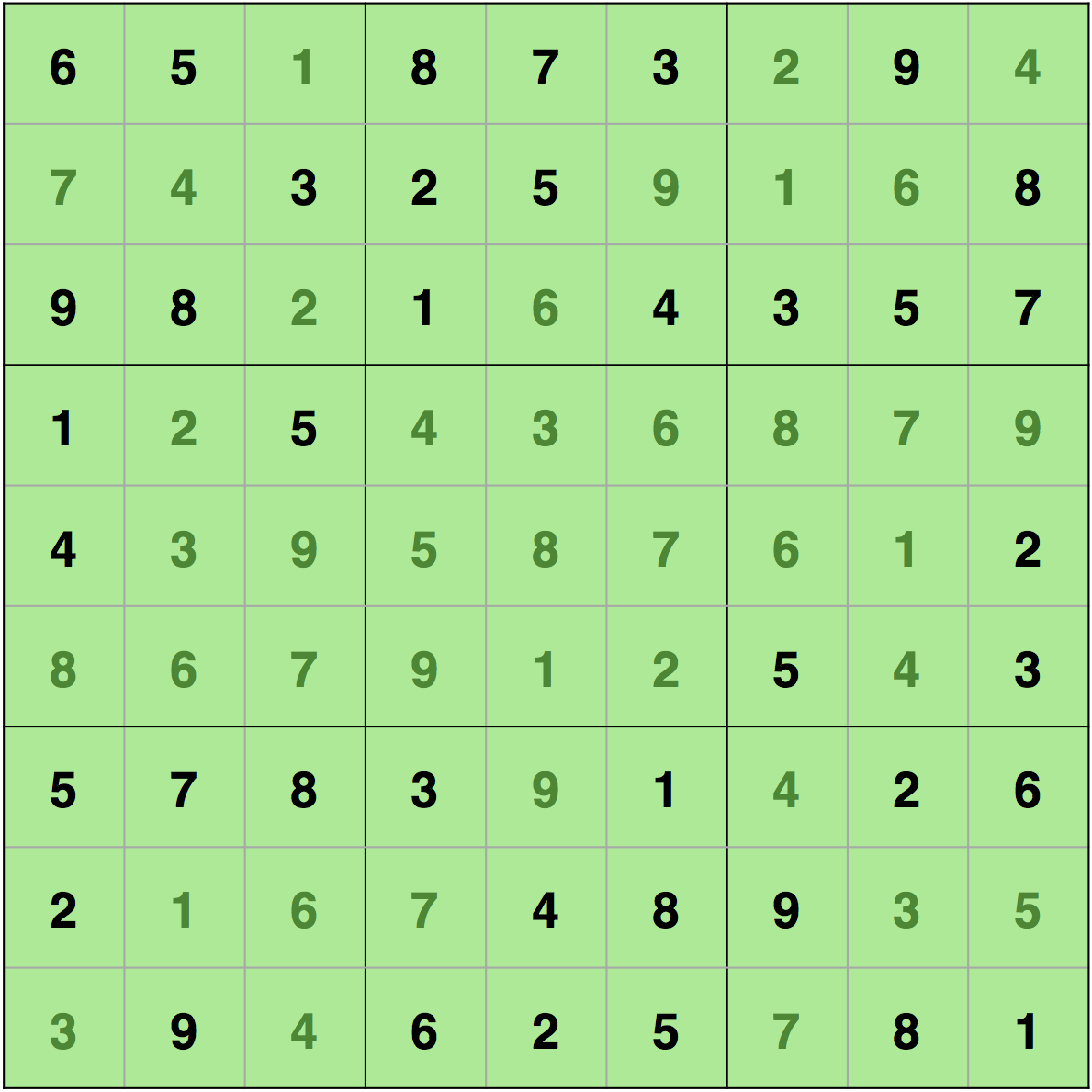In this post, we will introduce a Sudoku-solving algorithm using backtracking. If you don’t know about backtracking, then just get some basic knowledge from this article

Sudoku is a 9×9 matrix filled with numbers 1 to 9 in such a way that every row, column, and sub-matrix (3×3) has each of the digits from 1 to 9. We are presented with a partly filled 9×9 matrix and have to fill every remaining cell in it. For instance, a Sudoku problem is given below.And its solution is given beneath.You can see that every row, column, and sub-matrix (3×3) includes each digit from 1 to 9. Thus, we can also assume that a Sudoku is considered wrongly filled if it meets any of these criteria:

1. Any row contains the same number more than once.
2. Any column contains the same number more than once.
3. Any 3×3 sub-matrix has the same number more than once.

In backtracking, we first begin with a sub-solution and if this sub-solution doesn’t give us an accurate final answer, then we just come back and improve our sub-solution. We are going to solve our Sudoku in an alike way. The steps which we will follow are:

• If there are no unallocated cells, then the Sudoku is already solved. We will just return true.
• Or else, we will fill an unallocated cell with a digit between 1 to 9 so that there are no conflicts in any of the rows, columns, or the 3×3 sub-matrices.
• Now, we will try to fill the next unallocated cell and if this happens successfully, then we will return true.
• Else, we will come back and change the digit we used to fill the cell. If there is no digit which fulfills the need, then we will just return false as there is no solution to this Sudoku.

So, let’s code this up.

### C

```#include <stdio.h>

#define SIZE 9

//sudoku problem
int matrix = {
{6,5,0,8,7,3,0,9,0},
{0,0,3,2,5,0,0,0,8},
{9,8,0,1,0,4,3,5,7},
{1,0,5,0,0,0,0,0,0},
{4,0,0,0,0,0,0,0,2},
{0,0,0,0,0,0,5,0,3},
{5,7,8,3,0,1,0,2,6},
{2,0,0,0,4,8,9,0,0},
{0,9,0,6,2,5,0,8,1}
};

//function to print sudoku
void print_sudoku()
{
int i,j;
for(i=0;i<SIZE;i++)
{
for(j=0;j<SIZE;j++)
{
printf("%d\t",matrix[i][j]);
}
printf("\n\n");
}
}

//function to check if all cells are assigned or not
//if there is any unassigned cell
//then this function will change the values of
//row and col accordingly
int number_unassigned(int *row, int *col)
{
int num_unassign = 0;
int i,j;
for(i=0;i<SIZE;i++)
{
for(j=0;j<SIZE;j++)
{
//cell is unassigned
if(matrix[i][j] == 0)
{
//changing the values of row and col
*row = i;
*col = j;
//there is one or more unassigned cells
num_unassign = 1;
return num_unassign;
}
}
}
return num_unassign;
}

//function to check if we can put a
//value in a paticular cell or not
int is_safe(int n, int r, int c)
{
int i,j;
//checking in row
for(i=0;i<SIZE;i++)
{
//there is a cell with same value
if(matrix[r][i] == n)
return 0;
}
//checking column
for(i=0;i<SIZE;i++)
{
//there is a cell with the value equal to i
if(matrix[i][c] == n)
return 0;
}
//checking sub matrix
int row_start = (r/3)*3;
int col_start = (c/3)*3;
for(i=row_start;i<row_start+3;i++)
{
for(j=col_start;j<col_start+3;j++)
{
if(matrix[i][j]==n)
return 0;
}
}
return 1;
}

//function to solve sudoku
//using backtracking
int solve_sudoku()
{
int row;
int col;
//if all cells are assigned then the sudoku is already solved
//pass by reference because number_unassigned will change the values of row and col
if(number_unassigned(&row, &col) == 0)
return 1;
int n,i;
//number between 1 to 9
for(i=1;i<=SIZE;i++)
{
//if we can assign i to the cell or not
//the cell is matrix[row][col]
if(is_safe(i, row, col))
{
matrix[row][col] = i;
//backtracking
if(solve_sudoku())
return 1;
//if we can't proceed with this solution
//reassign the cell
matrix[row][col]=0;
}
}
return 0;
}

int main()
{
if (solve_sudoku())
print_sudoku();
else
printf("No solution\n");
return 0;
}
```

### Java

```class Sudoku
{

private static final int SIZE = 9;
private static int[][] matrix = {
{6,5,0,8,7,3,0,9,0},
{0,0,3,2,5,0,0,0,8},
{9,8,0,1,0,4,3,5,7},
{1,0,5,0,0,0,0,0,0},
{4,0,0,0,0,0,0,0,2},
{0,0,0,0,0,0,5,0,3},
{5,7,8,3,0,1,0,2,6},
{2,0,0,0,4,8,9,0,0},
{0,9,0,6,2,5,0,8,1}
};

private static void printSudoku()
{
for(int i=0;i<SIZE;i++)
{
for(int j=0;j<SIZE;j++)
{
System.out.print(matrix[i][j]+"\t");
}
System.out.println("");
}
}

//function to check if all cells are assigned or not
//if there is any unassigned cell
//then this function will change the values of
//row and col accordingly
private static int[] numberUnassigned(int row, int col)
{
int numunassign = 0;
for(int i=0;i<SIZE;i++)
{
for(int j=0;j<SIZE;j++)
{
//cell is unassigned
if(matrix[i][j] == 0)
{
//changing the values of row and col
row = i;
col = j;
//there is one or more unassigned cells
numunassign = 1;
int[] a = {numunassign, row, col};
return a;
}
}
}
int[] a = {numunassign, -1, -1};
return a;
}

//function to check if we can put a
//value in a paticular cell or not
private static boolean isSafe(int n, int r, int c)
{
//checking in row
for(int i=0;i<SIZE;i++)
{
//there is a cell with same value
if(matrix[r][i] == n)
return false;
}
//checking column
for(int i=0;i<SIZE;i++)
{
//there is a cell with the value equal to i
if(matrix[i][c] == n)
return false;
}
//checking sub matrix
int row_start = (r/3)*3;
int col_start = (c/3)*3;
for(int i=row_start;i<row_start+3;i++)
{
for(int j=col_start;j<col_start+3;j++)
{
if(matrix[i][j]==n)
return false;
}
}
return true;
}

//function to solve sudoku
//using backtracking
private static boolean solveSudoku()
{
int row=0;
int col=0;
int[] a = numberUnassigned(row, col);
//if all cells are assigned then the sudoku is already solved
//pass by reference because number_unassigned will change the values of row and col
if(a == 0)
return true;
//number between 1 to 9
row = a;
col = a;
for(int i=1;i<=SIZE;i++)
{
//if we can assign i to the cell or not
//the cell is matrix[row][col]
if(isSafe(i, row, col))
{
matrix[row][col] = i;
//backtracking
if(solveSudoku())
return true;
//if we can't proceed with this solution
//reassign the cell
matrix[row][col]=0;
}
}
return false;
}

public static void main(String[] args)
{
if (solveSudoku())
printSudoku();
else
System.out.println("No solution");
}
}
```

### Python

```SIZE = 9
#sudoku problem
#cells with value 0 are vacant cells
matrix = [
[6,5,0,8,7,3,0,9,0],
[0,0,3,2,5,0,0,0,8],
[9,8,0,1,0,4,3,5,7],
[1,0,5,0,0,0,0,0,0],
[4,0,0,0,0,0,0,0,2],
[0,0,0,0,0,0,5,0,3],
[5,7,8,3,0,1,0,2,6],
[2,0,0,0,4,8,9,0,0],
[0,9,0,6,2,5,0,8,1]]
#function to print sudoku
def print_sudoku():
for i in matrix:
print (i)

#function to check if all cells are assigned or not
#if there is any unassigned cell
#then this function will change the values of
#row and col accordingly
def number_unassigned(row, col):
num_unassign = 0
for i in range(0,SIZE):
for j in range (0,SIZE):
#cell is unassigned
if matrix[i][j] == 0:
row = i
col = j
num_unassign = 1
a = [row, col, num_unassign]
return a
a = [-1, -1, num_unassign]
return a
#function to check if we can put a
#value in a paticular cell or not
def is_safe(n, r, c):
#checking in row
for i in range(0,SIZE):
#there is a cell with same value
if matrix[r][i] == n:
return False
#checking in column
for i in range(0,SIZE):
#there is a cell with same value
if matrix[i][c] == n:
return False
row_start = (r//3)*3
col_start = (c//3)*3;
#checking submatrix
for i in range(row_start,row_start+3):
for j in range(col_start,col_start+3):
if matrix[i][j]==n:
return False
return True

#function to check if we can put a
#value in a paticular cell or not
def solve_sudoku():
row = 0
col = 0
#if all cells are assigned then the sudoku is already solved
#pass by reference because number_unassigned will change the values of row and col
a = number_unassigned(row, col)
if a == 0:
return True
row = a
col = a
#number between 1 to 9
for i in range(1,10):
#if we can assign i to the cell or not
#the cell is matrix[row][col]
if is_safe(i, row, col):
matrix[row][col] = i
#backtracking
if solve_sudoku():
return True
#f we can't proceed with this solution
#reassign the cell
matrix[row][col]=0
return False

if solve_sudoku():
print_sudoku()
else:
print("No solution")
```

## Explanation of the code

Initially, we are just making a matrix for Sudoku and filling its unallocated cells with 0. Thus, the matrix contains the Sudoku problem and the cells with value 0 are vacant cells.

`print_sudoku()` → This is just a function to print the matrix.

`number_unassigned` → This function finds a vacant cell and makes the variables ‘row’ and ‘col’ equal to indices of that cell. In C, we have used pointers to change the value of the variables (row, col) passed to this function (pass by reference). In Java and Python, we are returning an array (or list, for Python) which contains these values. So, this function tells us if there is any unallocated cell or not. And if there is any unallocated cell then this function also tells us the indices of that cell.

`is_safe(int n, ``int r``, int c)` → This function checks if we can put the value ‘n’ in the cell (r, c) or not. We are doing so by first checking if there is any cell in the row ‘r’ with the value ‘n’ or not – `if(matrix[r][i] == n)`. Then we are checking if there is any cell with the value ‘n’ in the column ‘c’ or not – `if(matrix[i][c] == n)`. And finally, we are checking for the sub-matrix. `(r/3)*3` gives us the starting index of the row r. For example, if the value of ‘r’ is 2 then it is in the sub-matrix which starts from (0, 0). Similarly, we are getting the value of starting column by `(c/3)*3`. Thus, if a cell is (2,2), then this cell will be in a sub-matrix which starts from (0,0) and we are getting this value by `(c/3)*3` and `(r/3)*3`. After getting the starting indices, we can easily iterate over the sub-matrix to check if we can put the value ‘n’ in that sub-matrix or not.
`solve_sudoku()` → This is the actual function that solves the Sudoku and uses backtracking. We are first checking if there is an unassigned cell or not by using the `number_unassigned` function and if there is no unassigned cell then the Sudoku is solved. `number_unassigned` function also gives us the indices of the vacant cell. Thus, if there is any vacant cell then we will try to fill this cell with a value between 1 to 9. And we will use the `is_safe` to check if we can fill a particular value in that cell or not. After finding a value, we will try to solve the rest of the Sudoku `solve_sudoku`. If this value fails to solve the rest, we will come back and try another value for this cell `matrix[row][col]=0;`. The loop will try other values in the cell.